Science Research
Volume 4, Issue 1, February 2016, Pages: 21-25

Graded SiNx/SiOxNy Layers as Antireflective Coatings for Solar Cells Based on GaAs and Silicon Crystalline

Ousmane Ba*, Nacire Mbengue, Fatou Dia, Alassane Diaw, Mamadou Moustapha Diop, Bassirou Ba, Fabé Idrissa Barro

Physical Department, Laboratory of the Semiconductors and Solar Energy (LASSE), University Cheikh Anta Diop, Dakar, Senegal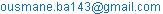(O. Ba)

Ousmane Ba, Nacire Mbengue, Fatou Dia, Alassane Diaw, Mamadou Moustapha Diop, Bassirou Ba, Fabé Idrissa Barro. Graded SiNx/SiOxNy Layers as Antireflective Coatings for Solar Cells Based on GaAs and Silicon Crystalline. Science Research. Vol. 4, No. 1, 2016, pp. 21-25. doi: 10.11648/j.sr.20160401.14

Abstract: These days solar cells layers are increasingly used primarily because of their low cost. During the last decades the performance of these cells were clearly improved. In the present work, a comparative study of two photovoltaic materials that are gallium arsenic and silicon. This allowed us to define the most efficient photovoltaic material. We have used for this purpose a method of adequate global search optimization for the search for optimal performance based on structural parameters (doping and thickness) of solar cells. This optimization method has allowed us to obtain a return of 26.15% for a solar cell based on GaAs and 18.15% for a solar cell based on Si, we were interested as to show the influence of the depth of penetration of light photons on the solar cells based on Si and GaAs which showed that the variation of the current density and more importantly on the deposit Arsenide Gallium 45.3 A / cm2.

Keywords: Solar Cells, GaAs, Si, Anti-Reflective Coating, Optimization, Performance

Contents

1. Introduction

Solar cells based on Gallium Arsenide and Silicon are considerable development efforts center because of their high conversion efficiency and the potential for improvement in performance.

Solar cells based on Ga and As have the highest efficiency measured (~ 25.7% at AM1.5 spectrum) of any single junction photovoltaic device. Solar cells based on GaAs have achieved high yields, in part because the energy gap of GaAs is close to the ideal value of 1.5 eV resulting in a maximum efficiency of photovoltaic conversion in a solar cell using single junction [1,2].

Further increases in efficiency are possible using superimposed junctions (tandem, triple, quadruple) and / or a concentrated lighting. Solar cells based on GaAs have additional significant advantages .

Compared with silicon solar cells, GaAs has electronic properties that are superior to those of silicon. The electron saturation speed is higher and their mobility is larger. With respect to silicon cells, another advantage of GaAs in that it has a direct energy gap, meaning that it can be used to emit light effectively.

Most improvements on solar cells based on silicon originates improving their structures (textured surfaces for the light trapping) and a surface passivation (SiO2 / interfaces if to reduce speed surface recombination) .

We will give a comparison and optimization of the efficiency of solar cells to gallium arsenide-based and silicon-based using the equations governing the solar cells that can be found in [5,6].

2. Theoretical Method

The Fig. 1 shows an incident electromagnetic wave at an interface and the reflected portion and the transmitted portion of this wave.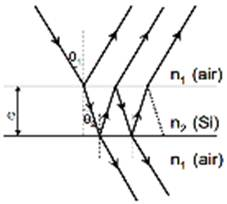Fig. 1. Model of an antireflection coating.

Using the Maxwell equations and boundary conditions  derives proven equations to the coefficients of reflection and transmission of the amplitude of the electromagnetic field to an interface.

The optical matrix approach is typically used to calculate the reflection coefficient. The relationship of matrix de fining the problem of two antireflection layers is given in reference .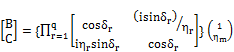(1)

where(2)

and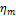: is the admittance.

The optical admittance is given by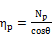(3)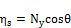(4)

B and C are the total amplitudes of the magnetic and electric fields of the incident light propagating in the medium. Thus, the optical admittance is given by the ratio

Y = C / B                   (5)

The following relationships give the reflectance, transmittance and absorption, respectively.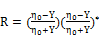(6)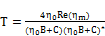(7)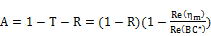(8)

Thus, each layer is represented by a 2 × 2 matrix M in the following form: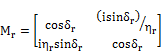(9)

For a photovoltaic (PV) cell, it is important to have a minimum reflection over the whole spectral range. The residual mean reflectance is a parameter used to characterize an anti-reflective layer. It is defined by :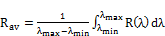(10)

where λmin, λmax and are the minimum and maximum values of the range of wavelengths, R is the reflectance. However, cell performance is influenced by other parameters such as photon flux F (λ) and the internal quantum efficiency IQE (λ) of the cell. The weighted reflectance of solar radiation or SWR (Solar Reflectance Weighted) is one of the most useful parameters for evaluating the performance of an anti-reflective layer in a PV cell. It is the ratio of the number of photons "useful" (likely to generate carriers) lost by reflection on the total number of "useful" photons. It is defined by [10,11]: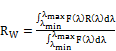(11)

Where F is the flow of incident photon and R reflectance. The photon flux is related to the sunlight I (λ) by the following relationship: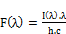(12)

Where h is Planck's constant and c is the speed of light. The solar radiation values can be found in . The spectral response of the silicon being in the range 300 - 1100 nm makes it possible to fix the limits of the integral in (9) and (10) these two values. The objective in optimizing an antiglare device for the PV cell is to maximize the short circuit current density JSC. The latter can be evaluated for a single-junction cell, using the formula: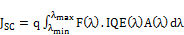(13)

Where q is the electron charge, F (λ) of the incident photon flux, IQE (λ) the internal quantum efficiency of the solar cell, A (λ) absorption by the silicon. The current densities associated with the photon flux reflected and transmitted can be calculated from equation (12) by substituting A (λ) by R (λ) or T (λ), respectively [12,13].

3. Results and Discussions

The figure shown the refractive index of Si and GaAs for different values of the wavelength λ (nm).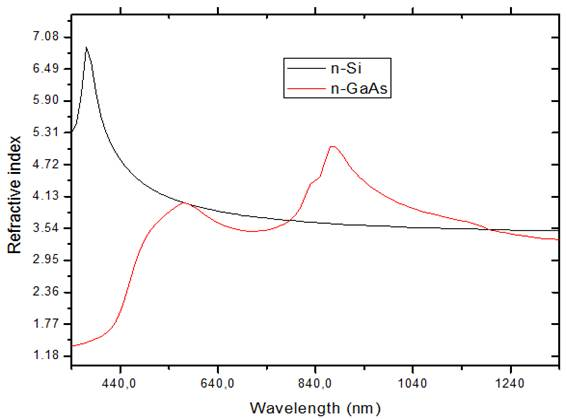Fig. 2. Refractive index of Si and GaAs as a function of wavelength (nm).

The refractive index of GaAs is little influenced by that of Si against undergoes an increase of 5, 31 6, 87 and then a decrease to 3, 50. The GaAs and because the surface recombination velocity high, which is of the order of 106 cm/s , the thickness of the layer must be reduced to a few hundredths of a micron.

In the Figure 3, there is shown the refractive index of Si/SiNx/SiOxNy of different wavelengths.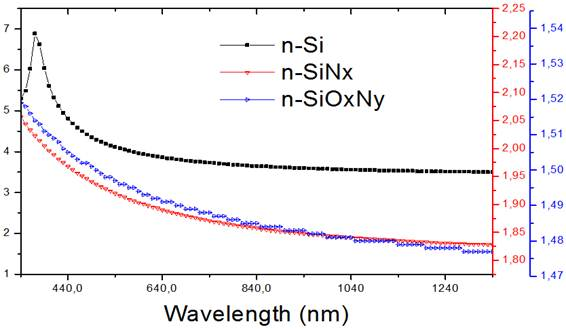Fig. 3. Refractive index of Si/ SiNx/SiOxNy as a function of wavelength (nm).

The effect of the refractive index on the wavelength is shown in Figure 3. It is noted that for wavelengths below 440 nm, the refractive indices of the layers decreases substantially with increasing the thickness, this phenomenon can be attributed to the absorption of light in the layers.

In the Fig. 4, there is shown the refractive index of SiNx/SiOxNy/GaAs values of different wavelengths.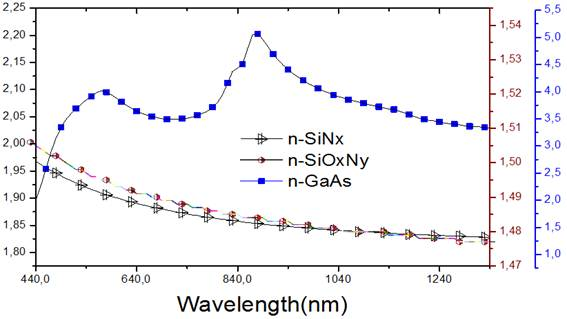Fig. 4. Refractive index of SiNx / SiOxNy / GaAs as a function of the wavelength.

The refractive index of SiNx and SiOxNy decreases slightly when the GaAs increases. This increase is insignificant since these materials are not a barrier to moisture and surface passivation as effectively providing that the materials such as SiNx and SiOxNy. By increasing the amount of Si in the SiOxNy, the refractive index increases, but the material becomes increasingly absorbing especially for smaller wavelengths.

3.1. Reflection and Transmission

The spectra of reflection and transmission of solar cells based on silicon are given in Fig. 5.

The maximum of the reflection band is near 540 nm for reflection according to the wavelength and 3 eV. The influence of the presence of a back passivation layer improves the intensity of the current density, providing better current, which greatly increases with the stack SiNx/SiOxNy/Si. This confirms the appropriate properties of the silicon nitride SiNx for use as layer anti-reflection in the solar cell.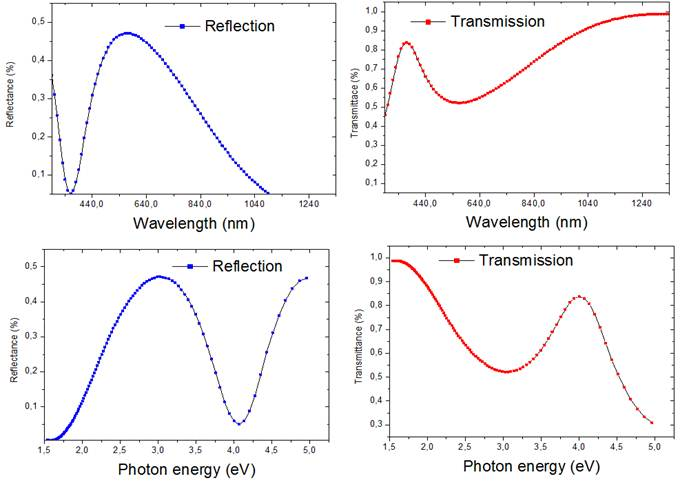Fig. 5. Reflection and transmission in function of the energy and the wavelength for a Si-based cell.

The spectra of reflection and transmission of solar cells to gallium arsenide base are given in Fig. 6.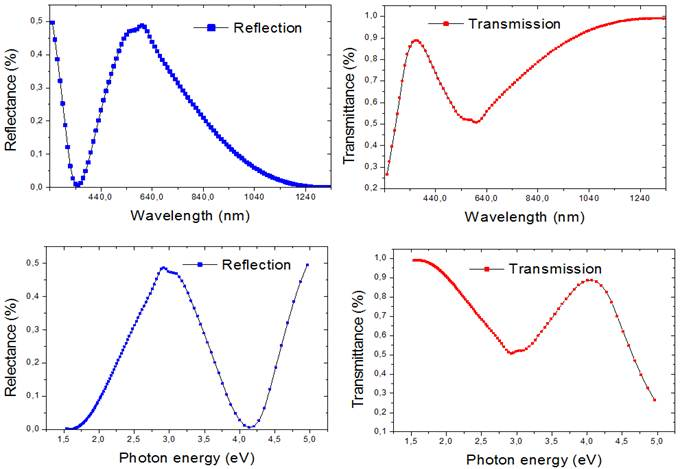Fig. 6. Reflection and transmission in function of the energy and the wavelength for a GaAs cell.

This fig. 6 clearly shows that the decrease of the reflection increases the yield, this is explained by the admissible current density decreases with the decrease in the rate of recombination, and therefore we obtain an increase of the potential barrier with the decrease in the rate of recombination. The high yields of the solar cell based on the GaAs-based cells compared to Si, are generally attributed to the reduction of the series resistance and the surface recombination velocity.

3.2. Short-Circuit Current Density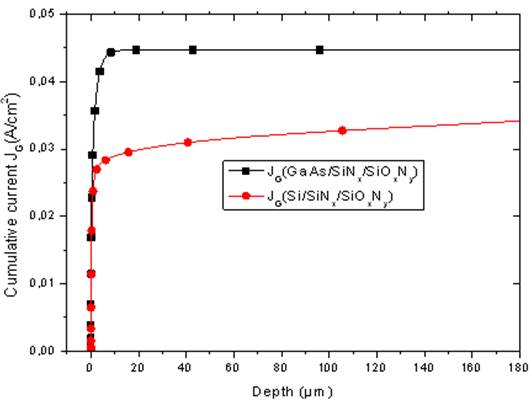Fig. 7. Variation of current density as a function of depth.

In order to visualize the importance of GaAs solar cells, we made a comparative study between two cells based on Si and GaAs. In Figure 6 is shown the characteristic J (V) as a function of depth for these two cells. It is noted that the GaAs cell gives a larger short-circuit current than Si.

4. Conclusion

In this work, we focused on the comparative study of two solar cells in one silicon Si, and the other based on gallium arsenide GaAs. We have seen an improvement in performance with a back passivation layer stacked thin oxide / silicon nitride / silicon oxide, where the yield is 10.77%. Solar cells based on GaAs give a small short-circuit current density, open circuit voltage greater and better performance as solar cells based on Si.

References

1. Aiken, D. J. (2000a). Antireflection coating design for series interconnected multi-junction solar cells. Progress in Photovoltaic: Research and Applications, volume 8, numéro 6, p. 563-570.
2. J. J. Liou and W. W. Wong, "Comparison and optimization of the performance of Si and GaAs solar cells", Solar Energy Mater. Solar Cells, 28(1992), pp. 9-28.
3. Bai Yiming, Chen Nuofu, Dai Ruixuan, et al.Dispersion effect on double-layer antireflection coatings of GaAs solar cells. Chinese Journal of Semi-conductors, 2006, 27(4): 725 (in Chinese).
4. J.-F. Lelièvre, E. Fourmond, A Kaminski, et al., Solar Energy Material & Solar Cells vol.93 (2009), 1281-1289.
5. Jaouad, A. et Aimez, V.(2006). Passivation of air-exposed AlGaAs using low frequency plasma-enhanced chemical vapor deposition of silicon nitride. Applied Physics Letters, volume 89, numéro 9, p. 092125.
6. H.E. Elgamel and M. Allen Barnett, ‘Efficient Combination of Surface and Bulk Passivation Schemes of High-efficiency Multicrystalline Silicon Solar Cells’, J. Appl. Phys. 78, 5, pp. 3457-3461, 1995.
7. Jaouad, A., Aimez, V. et Aktik, C.(2004). GaAs passivation by low-frequency plasma enhanced chemical vapour deposition of silicon nitride. Electronics Letters, volume 40, numéro 16, p. 1024-1026.
8. S. C. Tsaur et al., "Theoretical and experimental results for GaAs solar cells", in proceedings of the fourth international symposium on GaAs and related compounds, conference series N°17, The Institute of Physics, London, U.K.,(2009),pp.156.
9. G. Santana and A. Morales-Acevedo, Solar Energy Materials & Solar Cells 60 (2000) 135.
10. G Santana and A. Morales-Acevedo. Optimization of pecvd SiNx H films for silicon solar cells. Solar Energy Materials and Solar Cells, pages 135–142, 2000.
11. H. Mäckel, R Lüdeman. "Detailed study of the composition of hydrogenated SiNx layers for high-quality silicon surface passivation", Journal of Applied physics, 92(5), 2002, pp. 2602-2609.
12. M.I. Alayo, I. Pereyra, W.L. Scopel, M.C.A. Fanitini, "On the nitrogen and oxygen incorporation in plasma-enhanced chemical vapour deposition (PECVD) SiOxNy films", Thin Solid Films, 402, 2002, pp. 254-161.
13. J.J. Liou, "Physical Models for Predicting the Performances of Si/Si, AlGaAs/GaAs and Si/SiGe Solar Cells," Solar Energy Materials and Solar Cells, vol. 29, no. 3, (2009), pp. 261-276.
14. Sadek Khellaf and Abdelhamid Ounissi "Optimizing the Efficiency of Solar Cells based on GaAs University Center of Bechar, Algeria vol. 69, no. 2-3, (2005), pp. 57-63.

 Contents 1. 2. 3. 3.1. 3.2. 4.
Article ToolsAbstractPDF(1462K)